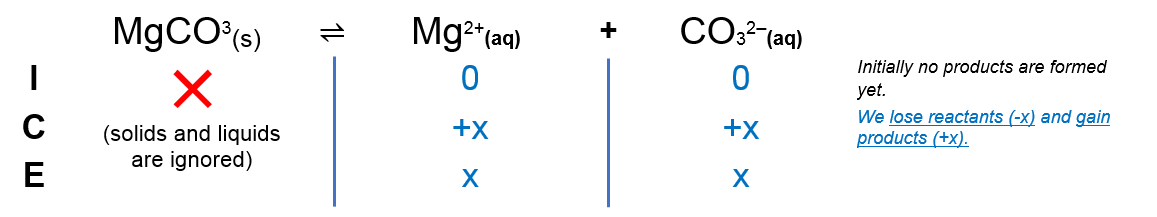# Problem: At 25 °C the solubility of magnesium carbonate is 3.16 x 10-3 mol/L. Calculate the value of Ksp at this temperature. Give your answer in scientific notation to 2 SIGNIFICANT FIGURES (even though this is strictly incorrect).

###### FREE Expert Solution

We’re being asked to determine Ksp of magnesium carbonate at 25 °C.

Recall that Ksp is an equilibrium constant related to slightly soluble ionic compounds

The dissociation of magnesium carbonate in solution is as follows:

MgCO3(s)  Mg2+(aq) + CO32–(aq)

We can construct an ICE table for this dissociationRemember that solids are ignored in the ICE table and Ksp expression.

85% (419 ratings)###### Problem Details

At 25 °C the solubility of magnesium carbonate is 3.16 x 10-3 mol/L. Calculate the value of Ksp at this temperature. Give your answer in scientific notation to 2 SIGNIFICANT FIGURES (even though this is strictly incorrect).